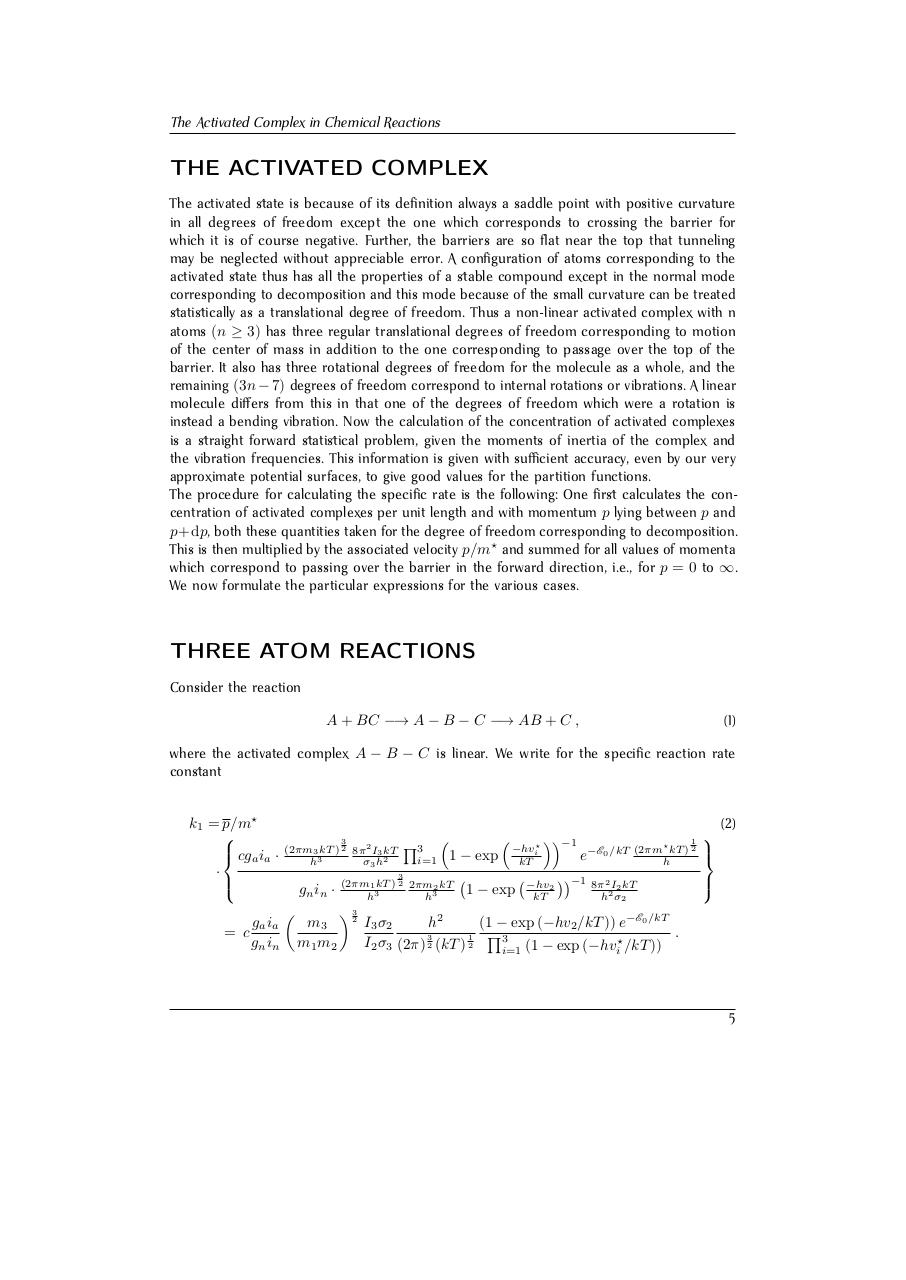# Henry Eyring. The Activated Complex in Chemi.pdfPage 1 2 3 4 5 6 7 8 9 10 11 12 13

#### Text preview

The Activated Complex in Chemical Reactions

THE ACTIVATED COMPLEX
The activated state is because of its definition always a saddle point with positive curvature
in all degrees of freedom except the one which corresponds to crossing the barrier for
which it is of course negative. Further, the barriers are so flat near the top that tunneling
may be neglected without appreciable error. A configuration of atoms corresponding to the
activated state thus has all the properties of a stable compound except in the normal mode
corresponding to decomposition and this mode because of the small curvature can be treated
statistically as a translational degree of freedom. Thus a non-linear activated complex with n
atoms (n ≥ 3) has three regular translational degrees of freedom corresponding to motion
of the center of mass in addition to the one corresponding to passage over the top of the
barrier. It also has three rotational degrees of freedom for the molecule as a whole, and the
remaining (3n − 7) degrees of freedom correspond to internal rotations or vibrations. A linear
molecule differs from this in that one of the degrees of freedom which were a rotation is
instead a bending vibration. Now the calculation of the concentration of activated complexes
is a straight forward statistical problem, given the moments of inertia of the complex and
the vibration frequencies. This information is given with sufficient accuracy, even by our very
approximate potential surfaces, to give good values for the partition functions.
The procedure for calculating the specific rate is the following: One first calculates the concentration of activated complexes per unit length and with momentum p lying between p and
p+dp, both these quantities taken for the degree of freedom corresponding to decomposition.
This is then multiplied by the associated velocity p/m? and summed for all values of momenta
which correspond to passing over the barrier in the forward direction, i.e., for p = 0 to ∞.
We now formulate the particular expressions for the various cases.

THREE ATOM REACTIONS
Consider the reaction
A + BC −→ A − B − C −→ AB + C ,

(1)

where the activated complex A − B − C is linear. We write for the specific reaction rate
constant
k1 = p/m?

 cga ia ·
·

ga ia
= c
gn in

(2)
3
(2πm3 kT ) 2
3
h

2

Q3

8π I3 kT
σ3 h2

i=1



1 − exp



−hvi?

3

gn in ·


m3
m1 m2

(2πm1 kT ) 2 2πm2 kT
h3
h3

 32

1 − exp

−1

kT
−hv2
kT

?

1
kT ) 2

e−E0 /kT (2πmh

−1

8π 2 I2 kT
h2 σ2

I3 σ2
h2
(1 − exp (−hv2 /kT )) e−E0 /kT
.
Q3
3
1
?
I2 σ3 (2π) 2 (kT ) 2
i=1 (1 − exp (−hvi /kT ))

5